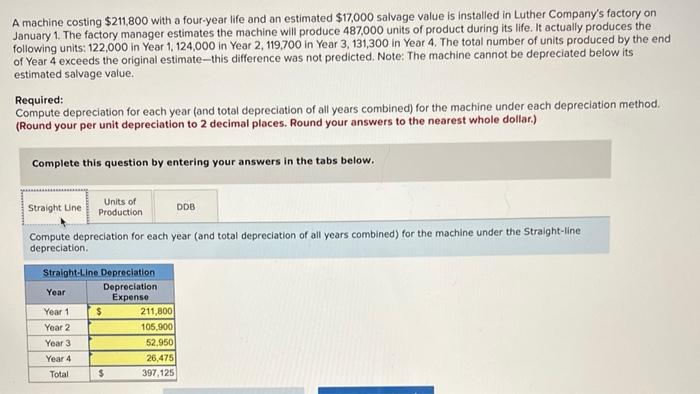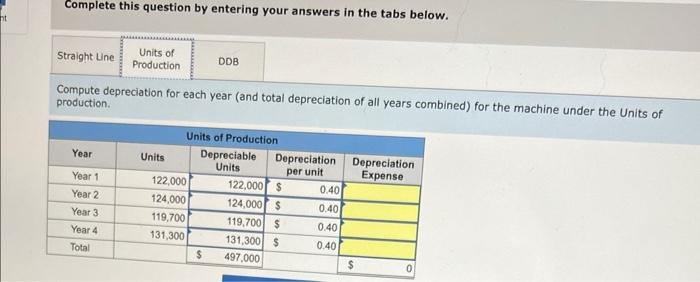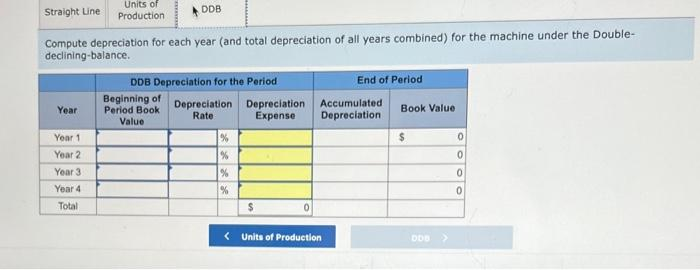Home / Expert Answers / Accounting / a-machine-costing-211-800-with-a-four-year-life-and-an-estimated-17-000-salvage-value-is-installe-pa947

# (Solved): A machine costing $211,800 with a four-year life and an estimated$17,000 salvage value is installe ...A machine costing with a four-year life and an estimated salvage value is installed in Luther Company's factory on January 1. The factory manager estimates the machine will produce 487,000 units of product during its life. It actually produces the following units: 122,000 in Year 1,124,000 in Year 2,119,700 in Year 3,131,300 in Year 4. The total number of units produced by the end of Year 4 exceeds the original estimate-this difference was not predicted. Note: The machine cannot be depreciated below its estimated salvage value. Required: Compute depreciation for each year (and total depreciation of all years combined) for the machine under each depreciation method. (Round your per unit depreciation to 2 decimal places. Round your answers to the nearest whole dollar.) Complete this question by entering your answers in the tabs below. Compute depreciation for each year (and total depreciation of all years combined) for the machine under the Straight-line depreciation. Complete this question by entering your answers in the tabs below. Compute depreciation for each year (and total depreciation of all years combined) for the machine under the Units of production. Compute depreciation for each year (and total depreciation of all years combined) for the machine under the Doubledeclining-balance.

We have an Answer from Expert